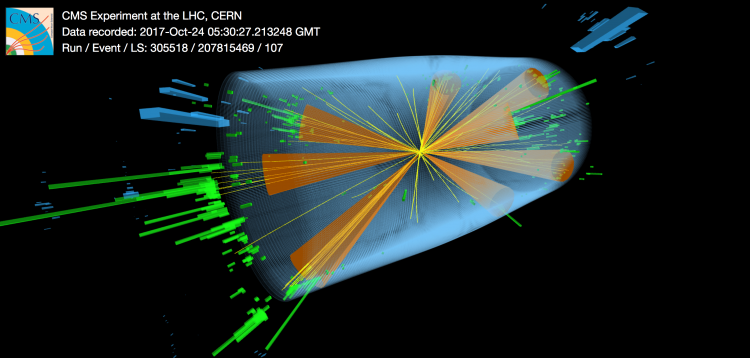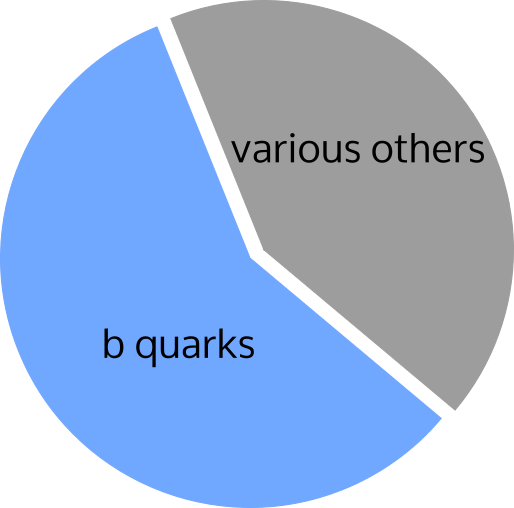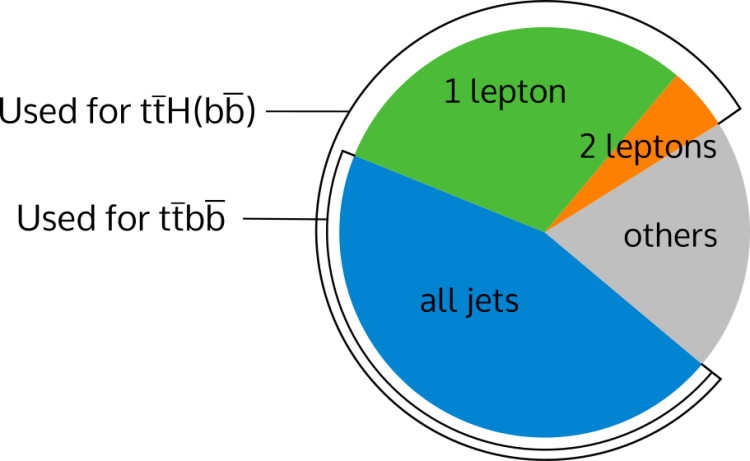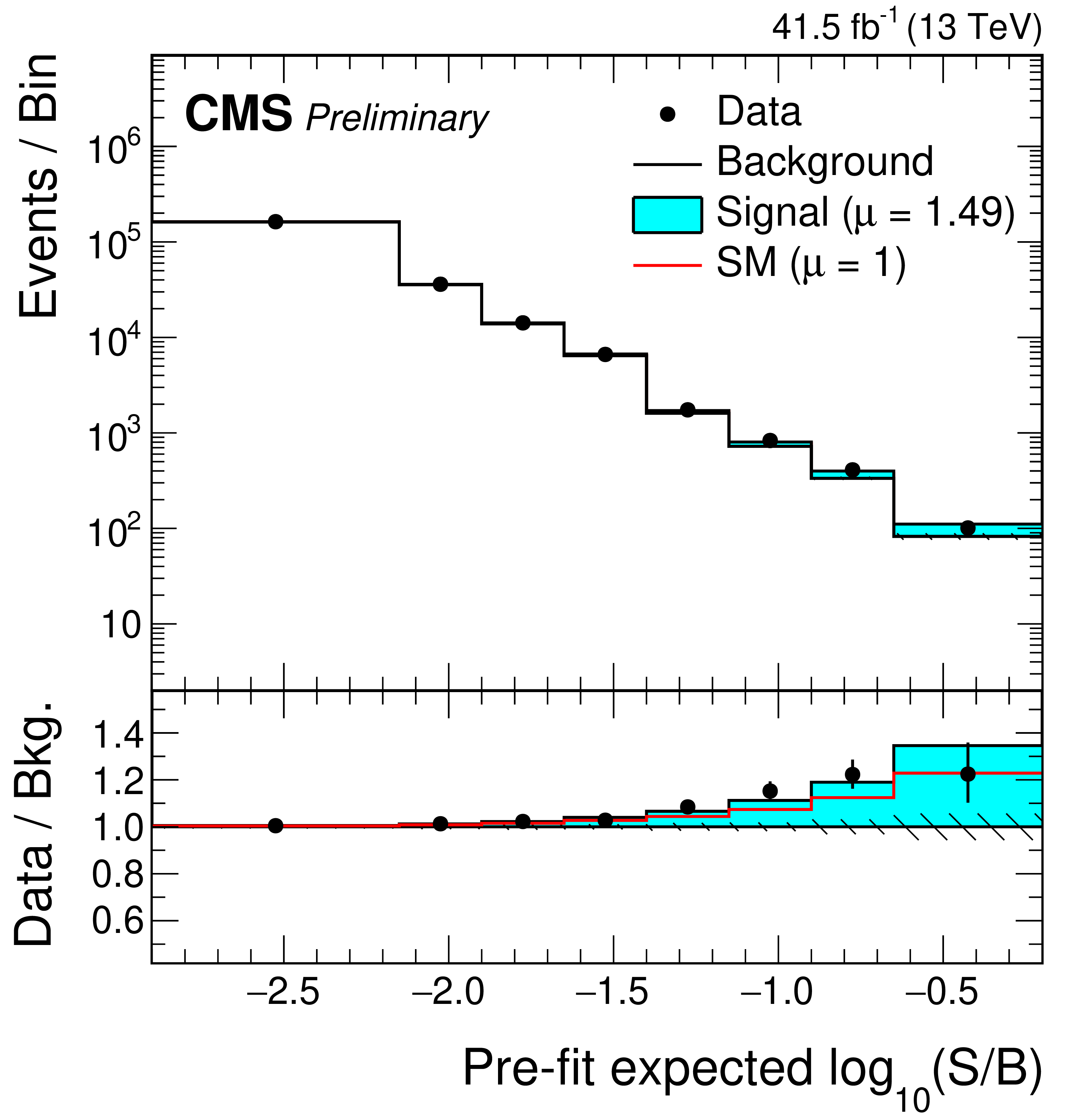•A collision where it is likely that a top quark-antiquark pair was produced together with two bottom quarks

•A collision where it is likely that a top quark-antiquark pair was produced together with two bottom quarks

•A collision where it is likely that a top quark-antiquark pair was produced together with two bottom quarks

•The top quark decay products can involve zero or one lepton (by which we mean an electron or a muon), resulting in different possible signatures when top quarks are produced in pairs. For the measurement of tt+bb we have used collisions where no lepton is present, which in itself is the most frequent possibility, whereas for the ttH (H→bb) analysis we have combined nearly all available decay channels.

•The Higgs boson decays most often into a pair of b quarks, which is why that decay mode was considered in this analysis.

The CMS collaboration has recently released new results on how the top quark and Higgs boson interact, and this required a much deeper understanding of the production of top quarks together with bottom quarks.

The two heaviest known elementary particles, the top (t) quark and the Higgs (H) boson, are deeply connected. They provide an essential probe of the Standard Model (SM) of particle physics, our best attempt so far at describing the fundamental particles and their interaction, and of hypothetical new physics beyond the SM. In the SM, the strength of the interaction between the Higgs boson and matter particles (quarks and leptons) is proportional to their mass. Since the top quark is the heaviest of all particles, the interaction between the Higgs boson and top quarks is also the strongest. As a result of that top-Higgs interaction, Higgs bosons can be produced in association with top quark pairs (this is called the ttH process and was first confirmed in 2018). Measuring the rate at which this rare process happens in the collisions between protons at the LHC, i.e. the probability that a given collision results in the simultaneous production of a top quark pair and a Higgs boson, is the most direct way to study the top-Higgs interaction. In turn, this tells us a lot about the nature of the Higgs boson and helps us answer questions such as: is the Higgs boson actually elementary? Are there other kinds of Higgs bosons out there? Is the universe stable?

Neither the Higgs boson nor the top quark can be observed directly with the CMS detector, unfortunately, since they are unstable and disintegrate (decay) nearly instantly to other particles. It is only indirectly, by observing the particles emerging out of their decays, that we can learn about the Higgs boson, the top quark, and their relationship. Because the Higgs boson is lighter than the top quark, it cannot decay to top quarks, and as a result, the majority of the produced Higgs bosons decay to a pair of the next-heaviest quark, the bottom (b) quark. This makes the H→bb decay mode a robust handle to study the rare ttH process (see Fig. 1). As for the top quark, its decay can, for example, involve a b quark and a charged lepton (typically only the electron or its heavier cousin the muon) and a neutrino, or a b quark and a pair of lighter quarks, such as those that make up the proton (the up and down quark). The story is the same for the top antiquark, so that when a top quark-antiquark pair is produced, we may end up with either zero, one or two charged leptons. While the CMS detector can directly measure electrons or muons, quarks will manifest themselves as "jets" of particles which we can detect and whose properties inform us about the original quarks. In particular, the relatively long lifetime of B hadrons, which are present in jets originating from b quarks, can be used to identify these jets from the jets produced by lighter quarks. In a new analysis of the data collected during 2017, CMS has studied the ttH (H→bb) process in nearly all the possible decay modes of the top quark pairs: with either zero, one, or two charged leptons, where the leptons can be electrons or muons (see Fig. 2). That challenging measurement has been carried out by several physicists from different institutes, who have joined forces to isolate this rare signal.Figure 1: The Higgs boson decays most often into a pair of b quarks, which is why that decay mode was considered in this analysis.

Unfortunately, ttH with H→bb is not the only way in which we may end up with a pair of top quarks and a pair of b quarks in the CMS detector. In about 95% of the collisions where b quarks are seen together with a top quark-antiquark pair, they do not originate from Higgs boson decays but are instead produced via a different mechanism: the strong interaction (QCD).  These Higgs-less tt+bb collisions create an important background with a signature in the detector that is almost identical to the coveted signal. Only by comparing in detail the predictions for these backgrounds with the actual data can we tell if ttH production is happening in the collisions, and at which rate. Thus, the tt+bb process needs to be correctly modelled and understood if we want to have any chance of measuring ttH (H→bb)! That is a challenging task, and independent measurements of the tt+bb production rate are essential to improve our understanding of this process. CMS has recently carried out that measurement using data collected in 2016, focusing on the scenario where the top quarks decay to quarks, so the collision contains only jets and no electron or muon (see Fig. 2). That measurement is, in turn, also made difficult because of the large background of events that do not contain top quarks but still multiple jets produced through the strong interaction.Figure 2: The top quark decay products can involve zero or one lepton (by which we mean an electron or a muon), resulting in different possible signatures when top quarks are produced in pairs. For the measurement of tt+bb we have used collisions where no lepton is present, which in itself is the most frequent possibility, whereas for the ttH (Hbb) analysis we have combined nearly all available decay channels.

Several advanced data analysis methods, relying heavily on machine learning techniques such as artificial neural networks, had to be used to make the processes in which we are interested distinguishable from the enormous backgrounds. A crucial technique among them is the identification, with reasonable confidence, of jets that are initiated by b quarks (b tagging). While b tagging helps in reducing the backgrounds, it is not sufficient to isolate the signal. By harnessing the subtle differences in the properties (particle energies and direction) of the signal and background processes, we have built algorithms that can tell us whether a particular collision is likely to be due to the signal we are after, or rather due to background processes (see Fig. 3). Separating the signal from the backgrounds in that way is crucial to increase the precision of our measurements!Figure 3: Collision yields in the ttH analysis as a function of the expected ratio of signal (S) and background (B) yields in the bins of the multivariate discriminant distributions used in the analysis (for this plot, several discriminant bins have been combined). The number of collisions observed in data (black markers) is compared to the background expectation (black line) and the extracted signal contribution (blue area); also shown is the signal contribution expected in the SM (red line).

With these new results we have measured the rate (also called "cross-section") of tt+bb with a precision of approximately 25%, and found it to be slightly larger than the theoretical predictions (see Fig. 4), indicating that the modelling of the tt+bb process can and should still be improved, and that additional measurements of the properties of the tt+bb process are needed. The observed ttH (H→bb) signal is compatible with our theoretical expectations and corresponds to a statistical significance of 3.9 standard deviations. This means that the probability that the observed signal is due to a fluctuation in the background processes is smaller than 0.005%! The results mark an essential step in improving our understanding of the properties of the Higgs boson and the top quark and constitute another test passed by the SM of particle physics.Figure 4: Comparison of the measured tt+bb cross-section (i.e. the rate, black line with green bands) with many theoretical predictions (black dots with error bars). The green bands show the uncertainty in the measurement, while the blue bars indicate the uncertainty in the theoretical predictions.